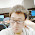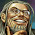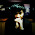## Longest Consecutive Sequence

Given an unsorted array of integers, find the length of the longest consecutive elements sequence.
For example,
Given [100, 4, 200, 1, 3, 2],
The longest consecutive elements sequence is [1, 2, 3, 4]. Return its length: 4.
Your algorithm should run in O(n) complexity.

### Analysis:

At first glance, the "longest" requirement may lead us to DP. But this problem actually tests data structure rather than a certain algorithm.

If there is no O(n) requirement, we can just sort the array (O(n log n)), then find then longest sequence after a scan.

With the time limit O(n), first of all, what comes to my mind is HASH MAP! Because hash map searches value by key in O(1) time. Note that in C++ STL, the map<> data structure is implemented by BST, so the insert in O(log n), therefore we need to use the unordered_map<>, which has O(1) time complexity.

Firstly, we put all the element into a map.
Secondly, how to find the consecutive elements? Since the O(n) requirement, scan the array is a must.
For each element, we need to find its consecutive elements. Consecutive means +1 or -1, a while loop is enough to handle. Search two directions respectively (+1, -1),  during the search if the key is found, remove the current item in the map. This is because if two items are consecutive, the longest elements for this two are the same, no need to search again. In this way, the length of longest consecutive elements can be easily found.

Note that in C++ map<>, find(key) function will return  the end() iterator if key does not exist, But if we use (mp[key]==false), when key is not in the map, the program will insert the key into the map with a default value, so use find function is a safer way.

### Code (C++):

class Solution {
public:

int longestConsecutive(vector<int> &num) {
// Start typing your C/C++ solution below
// DO NOT write int main() function
unordered_map<int,bool>mp;
for (int i=0;i<num.size();i++){
mp[num[i]]=true;
}

int res=0;
for (int i=0;i<num.size();i++){
int mx=1;
int fd = num[i];

mp.erase(num[i]);
while (mp.find(fd+1)!=mp.end()){
mx++;
mp.erase(fd+1);
fd++;
}

fd = num[i];
while (mp.find(fd-1)!=mp.end()){
mx++;
mp.erase(fd-1);
fd--;
}

if (mx>res){res=mx;}
}

return res;
}
};


### Code(Python):

class Solution:
# @param num, a list of integer
# @return an integer
def longestConsecutive(self, num):
dic = {}
maxlen = 1
for n in num:
dic[n] = 1
for n in num:
if dic.has_key(n):
tmp = n + 1
l = 1
while dic.has_key(tmp):
l+=1
del dic[tmp]
tmp+=1
tmp = n - 1
while dic.has_key(tmp):
l+=1
del dic[tmp]
tmp-=1
maxlen = max(l, maxlen)
else:
continue
return maxlen


1.but i hope the complexity of this algorithm is O(n^2)... the outer for loop runs 'n' times and inner while loop can run for atmost 'n' times..correct me if i am wrong

1.I think we can understand in this way, every time the consecutive element is find, the erase method is performed in the map. e.g. the [100,4,200,1,3,2] sequence, when scanning to "4", 3,2,1 are found in the while loop, and also 3,2,1 are eliminated from the map, thus when for loop goes to 1, or 3, or 2, the while loop actually runs O(1)and the condition fails then skip the while. In this way, the overall complexity is still O(n), isn't it?

2.I think in the first piece of code, after line 13, you can add one more line code

if(mp.find(num[i]) == mp.end()) continue;

This will make the code more obviously that the running time is O(n), and more correct.

3.what if consecutive length is 1? no elements are consecutive in the array, then the map items won't be eliminated, the worst case is still O(n^2). is it? i'm still confused with the O(n) requirement in this question, after reading multiple solutions online, the most straight forward solution to this question is like what you provided here, but i do think the time complexity is O(n^2). if it passed on leetcode, then the question itself is not correct, right? thank you!!

4.but insertion has worst time complexity of O(size of container)...then won't it become O(n^2)

2.Insertion in map takes O(log n). Since n insertions are made, the time complexity is actually O(n log n)

3.Actually this problem needs some data structure like union-find-set. That will led to a O(n) complexity.

4.Use unordered_set instead of map, that will lead to a O(n) solution.

5.Nice code in C#. Complexiety O(n)

http://onestopinterviewprep.blogspot.com/2014/04/longest-consecutive-sequence-of-numbers.html

6.You actually don't need a map here. If you need linear search complexity, use unordered_set will be enough here.

7.Use Radix sort O(kN) where k is # of digits in the max number

8.I have a solution:

public class Solution {
public int longestConsecutive(int[] num) {
int longest = 0;
Map map = new HashMap();
for(int i = 0; i< num.length; i++){
map.put(num[i], false);
}

int l, k;
for(int i = 0;i < num.length;i++){

if(map.containsKey(num[i]-1) || map.get(num[i])) continue;
map.put(num[i], true);
l = 0; k = num[i];
while (map.containsKey(k)){
l++;
k++;
}
if(longest < l) longest = l;

}
return longest;
}
}

There are other solutions here: http://traceformula.blogspot.com/2015/07/longest-consecutive-sequence-some.html

9.I have a solution:

public class Solution {
public int longestConsecutive(int[] num) {
int longest = 0;
Map map = new HashMap();
for(int i = 0; i< num.length; i++){
map.put(num[i], false);
}

int l, k;
for(int i = 0;i < num.length;i++){

if(map.containsKey(num[i]-1) || map.get(num[i])) continue;
map.put(num[i], true);
l = 0; k = num[i];
while (map.containsKey(k)){
l++;
k++;
}
if(longest < l) longest = l;

}
return longest;
}
}

There are other solutions here: http://traceformula.blogspot.com/2015/07/longest-consecutive-sequence-some.html